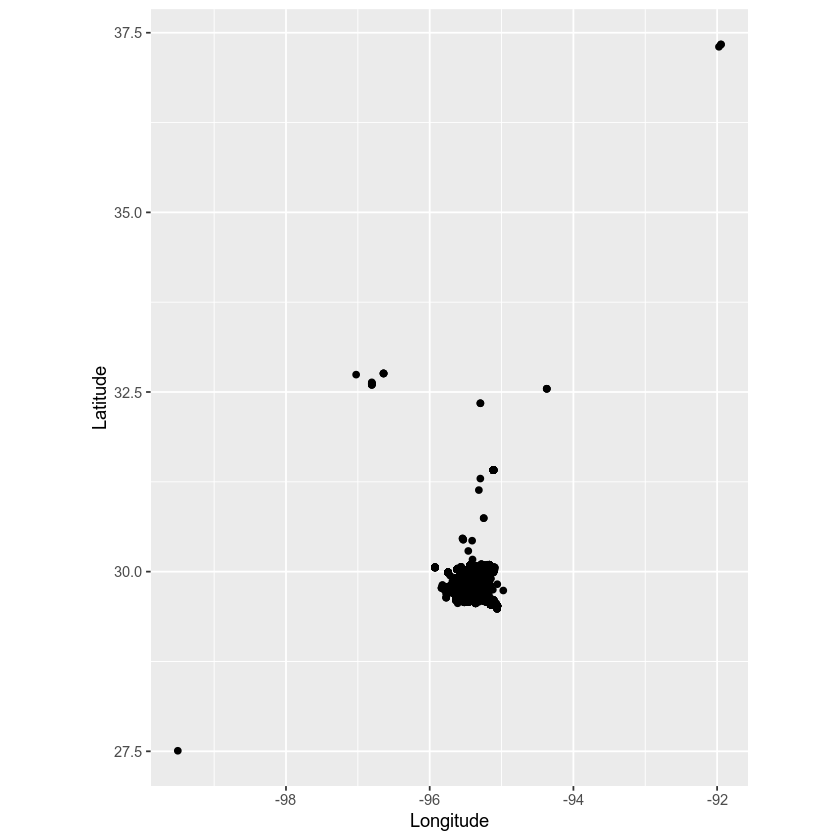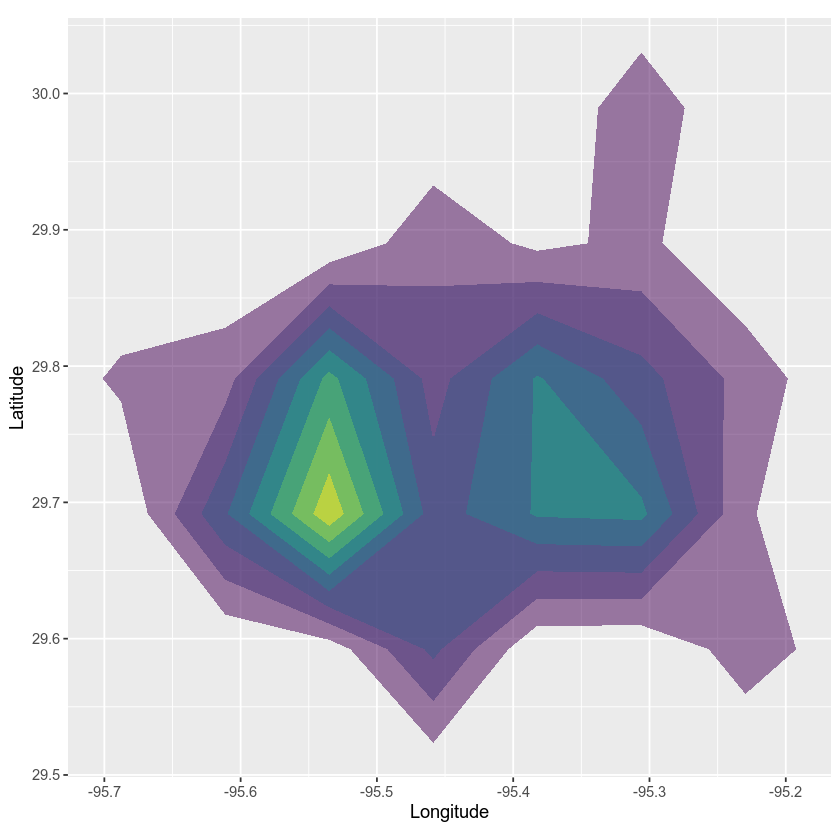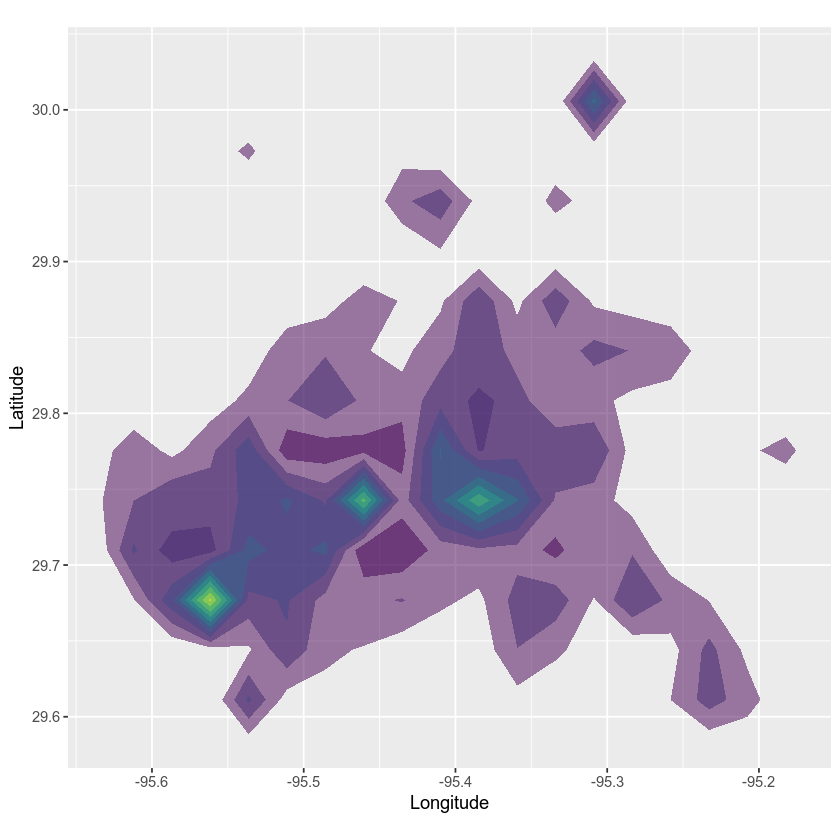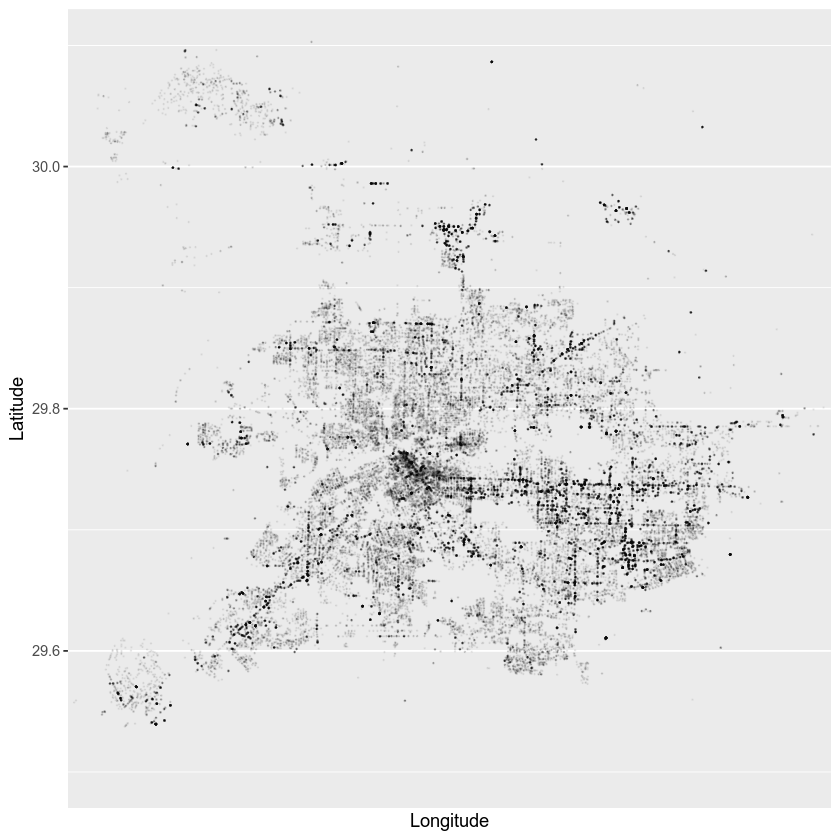# Computing and plotting 2d spatial point density in R

It is often useful to quickly compute a measure of point density and show it on a map. In this tutorial, we’ll demonstrate this using crime data from Houston, Texas contained in the ggmap R package.

## Objectives

• Compute 2d spatial density of points
• Plot the density surface with ggplot2

## Dependencies

• ggplot2
• ggmap

We’ll start by loading libraries. Note the ggmap package is no longer used in this lesson to generate a basemap, due changes in the way that maps are served from Google, but the data used in this tutorial are contained in the ggmap package.

library(ggplot2)
library(ggmap)


Then, we can load a built-in crime dataset for Houston, Texas.

data(crime)

# remove any rows with missing data
crime <- crime[complete.cases(crime), ]

# look at the structure of the crime data
str(crime)
## 'data.frame':	81803 obs. of  17 variables:
##  $time : POSIXct, format: "2010-01-01 06:00:00" "2010-01-01 06:00:00" ... ##$ date    : chr  "1/1/2010" "1/1/2010" "1/1/2010" "1/1/2010" ...
##  $hour : int 0 0 0 0 0 0 0 0 0 0 ... ##$ premise : chr  "18A" "13R" "20R" "20R" ...
##  $offense : Factor w/ 7 levels "aggravated assault",..: 4 6 1 1 1 3 3 3 3 3 ... ##$ beat    : chr  "15E30" "13D10" "16E20" "2A30" ...
##  $block : chr "9600-9699" "4700-4799" "5000-5099" "1000-1099" ... ##$ street  : chr  "marlive" "telephone" "wickview" "ashland" ...
##  $type : chr "ln" "rd" "ln" "st" ... ##$ suffix  : chr  "-" "-" "-" "-" ...
##  $number : int 1 1 1 1 1 1 1 1 1 1 ... ##$ month   : Ord.factor w/ 8 levels "january"<"february"<..: 1 1 1 1 1 1 1 1 1 1 ...
##  $day : Ord.factor w/ 7 levels "monday"<"tuesday"<..: 5 5 5 5 5 5 5 5 5 5 ... ##$ location: chr  "apartment parking lot" "road / street / sidewalk" "residence / house" "residence / house" ...
##  $address : chr "9650 marlive ln" "4750 telephone rd" "5050 wickview ln" "1050 ashland st" ... ##$ lon     : num  -95.4 -95.3 -95.5 -95.4 -95.4 ...
##  \$ lat     : num  29.7 29.7 29.6 29.8 29.7 ...


Let’s plot the locations of crimes with ggplot2.

ggplot(crime, aes(x = lon, y = lat)) +
geom_point() +
coord_equal() +
xlab('Longitude') +
ylab('Latitude')There seems to be a fair bit of overplotting.

Let’s instead plot a density estimate. There are many ways to compute densities, and if the mechanics of density estimation are important for your application, it is worth investigating packages that specialize in point pattern analysis (e.g., spatstat). If on the other hand, you’re lookng for a quick and dirty implementation for the purposes of exploratory data analysis, you can also use ggplot’s stat_density2d, which uses MASS::kde2d on the backend to estimate the density using a bivariate normal kernel.

ggplot(crime, aes(x = lon, y = lat)) +
coord_equal() +
xlab('Longitude') +
ylab('Latitude') +
stat_density2d(aes(fill = ..level..), alpha = .5,
geom = "polygon", data = crime) +
scale_fill_viridis_c() +
theme(legend.position = 'none')You can pass arguments for kde2d through the call to stat_density2d. In this case, we alter the argument h, which is a bandwidth parameter related to the spatial range or smoothness of the density estimate.

ggplot(crime, aes(x = lon, y = lat)) +
coord_equal() +
xlab('Longitude') +
ylab('Latitude') +
stat_density2d(aes(fill = ..level..), alpha = .5,
h = .02, n = 300,
geom = "polygon", data = crime) +
scale_fill_viridis_c() +
theme(legend.position = 'none')As an alternative, we might consider plotting the raw data points with alpha transparency so that we can see the actual data, not just a model of the data. We will also set coordinates to use as limits to focus in on downtown Houston.

ggplot(crime, aes(x = lon, y = lat)) +
geom_point(size = 0.1, alpha = 0.05) +
coord_equal() +
xlab('Longitude') +
ylab('Latitude') +
coord_cartesian(xlim = c(-95.1, -95.7),
ylim = c(29.5, 30.1))Updated: